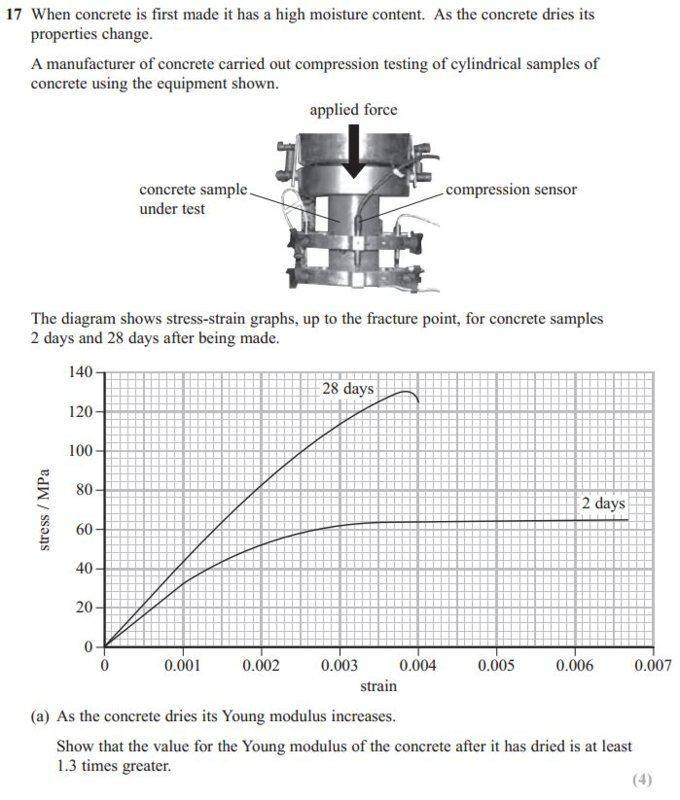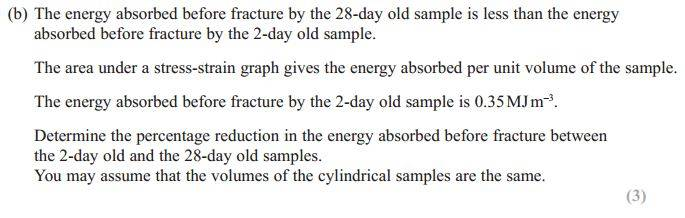# Young's Modulus, Stress/Strain graph and Calculating Energy

Homework Statement:
as below
Relevant Equations:
as belowFor part (b), I counted the number of squares underneath the 2 day sample to be roughly 33 'whole' squares (the 5by5 tiny squares is 1 whole sqaure).

I then equated 33 whole square = 0.35 MJ to calculate 1 whole square to be 0.0106 MJ.

I then counted the number of whole squares underneath the 28 day sample to roughly be 30.5 so the total absorbed energy is (30.5)(0.0106)=0.323..MJ

And found the percentage reduction to be around 7.6%. But then answer scheme has it between 12% and 15%.

No matter how many times I count the squares I can't get close to their answer. What am I doing wrong?

I know I could estimate the area by using the area of trapezium and triangle rules but I want to get this simple method right first.

•